﻿ Digital Circuits and Systems - Circuits i Sistemes Digitals (CSD) - EETAC - UPCBachelor's Degree in Telecommunications Systems and in Network EngineeringLecture 3 L3.3: Designing logic functions using plan C2: MoD, MoM   [P3] Method of decoders (MoD) and method of multiplexers (MoM). [17/3]

1.5.2.4. Plan C2: hierarchical design multiple-file (components and signals)

Since now we have studied several stratergies to implement logic functions based on canonical equations (maxterms or minterms), minimised equations (SoP or PoS), only-NOR or only-NAND transformations, or even any combination of equations. All of them related to a given circuit structure or plan A. Other methods are related to plan B: capturing the truth table or interpreeting it as a flowchart.

1.5.2.4.2. Method of decoders (MoD)

Now, we will implement logic circuits using hierarchical structures plan C2 based on decoder components. Decoders are standard logic components explained in the properties of which makes simple the implementation of logic functions.

How does the method of decoders for implementing logic functions work?

Example design: Circuit_K in Fig. 1 using the MoD.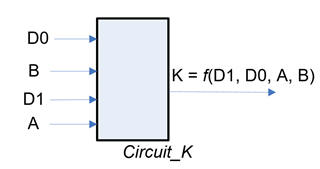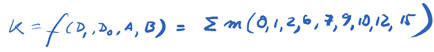Fig. 1. Circuit_K symbol nd truth table.

Fig. 2 shows how to invent the internal architecture based on MoD. Four inputs means using a Dec_4_16.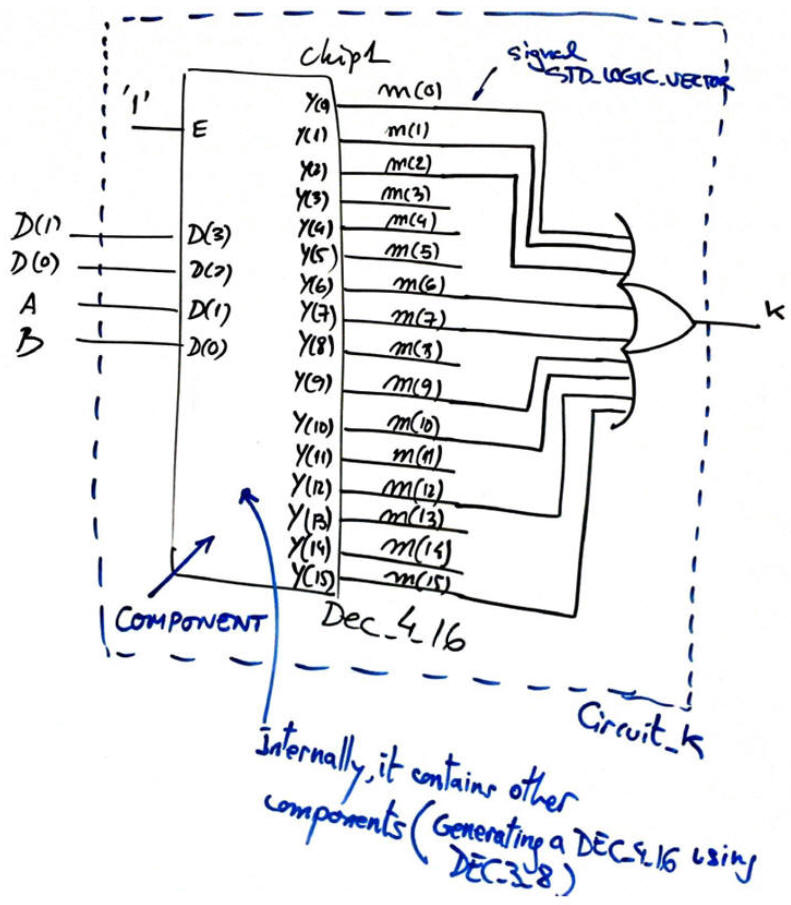Fig. 2. Circuit_K schematic using MoD.

As an example on how many options are available for building blocks, Fig. 3 shows how to build a Dec_4_16 using components Dec_3_8, this is applying plan C2 expandability. Chips Dec_3_8 at the same time can be composed of smaller blocs of the same kind such Dec_1_2, or instead be build using  single-file plan A (equations) or plan B (flowchart). As shown in the truth table, extra logic is required to enable only one internal Dec_3_8 at a time, or disable both of them when E = 0.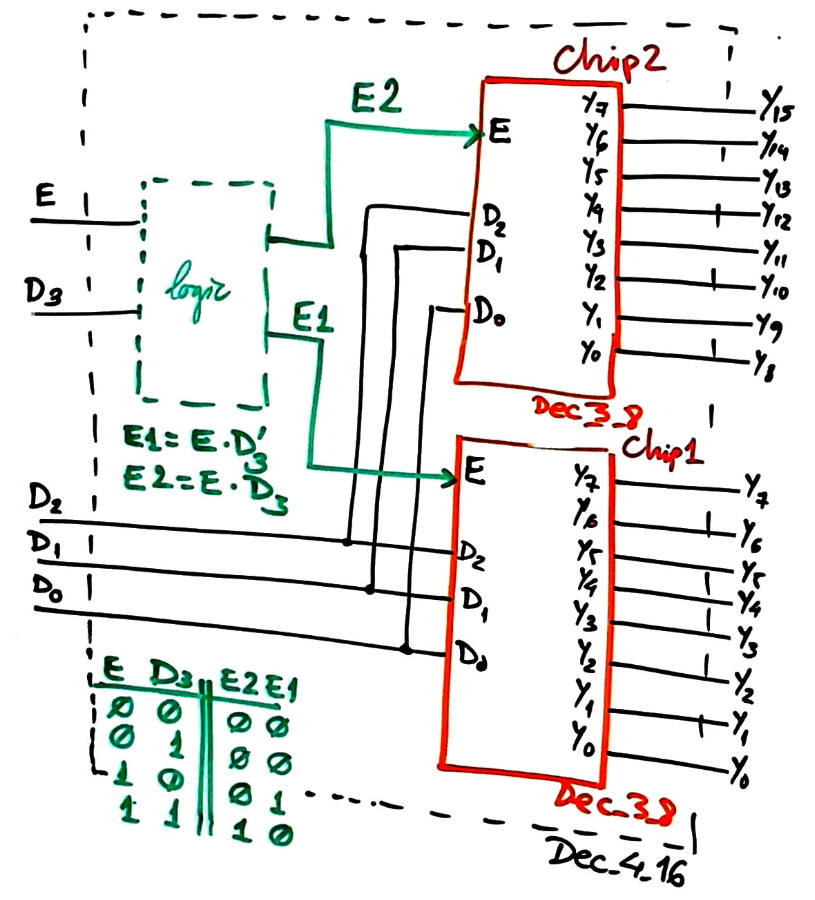Fig. 3. Circuit expandability: Building a Dec_4_16 using Dec_3_8 implemented using the plan B represented in Fig. 4.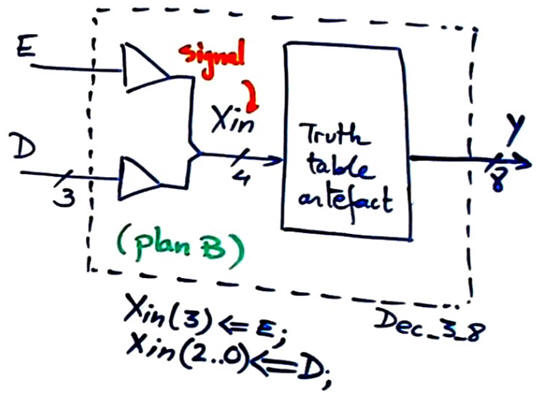Fig. 4. Creating Dec_3_8 using plan B.

Translating the schematic into VHDL generates the following files: Circuit_K.vhd, Dec_4_16.vhd, Dec_3_8.vhd.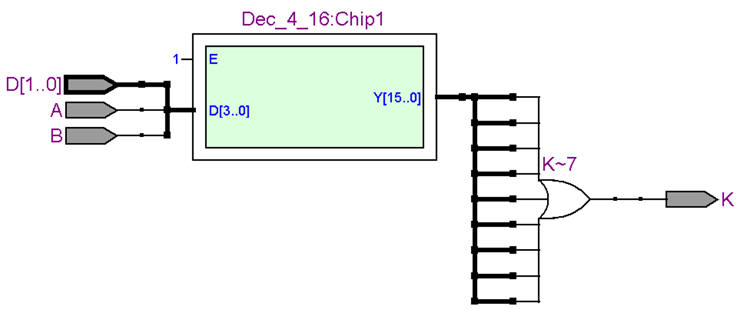Fig. 5. Circuit_K RTL view.

Using the same testbech from P1 tutorial Circuit_K_tb.vhd, we can verify our complete hierarchical structure as shown in Fig. 6.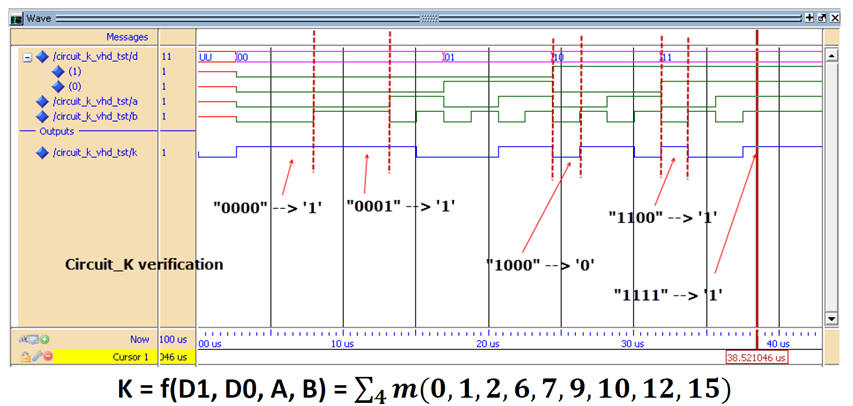Fig. 6. Circuit_K functional simulation and results.

Example design: Circuit_C using MoD.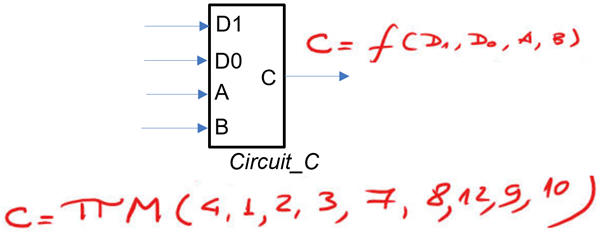Fig. 7. Circuit_C symbol and truth table.

Hierarchical structure Circuit_C: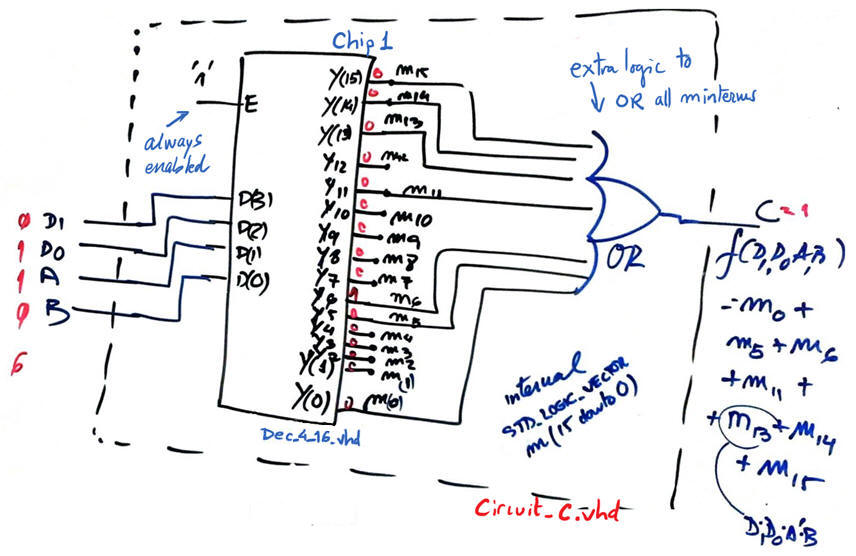Fig. 8. Circuit_C hierarchical structure using a decoder component.

1.5.2.4.3. Method of multiplexers  (MoM)

Multiplexers are standard logic components explained in .  How does  the method of multiplexers for implementing logic functions work?

A) Draw the symbol and expand the truth table to show all combinations

B) Section the truth table accordingly to the number of MUX channels

C) Connect the selection inputs to circuit inputs assigning inputs from from left to right.

D) Solve the remaining combinational logic that has to be attached to each channel

Example design: Comb_circuit using MoM and MUX_8, MUX_4 and MUX_2.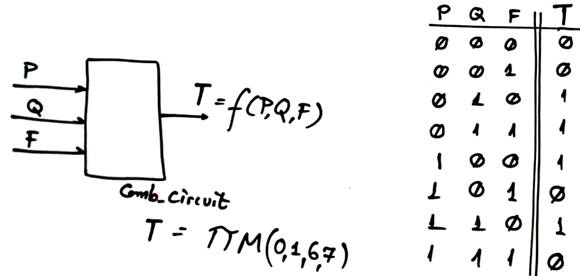Fig. 9. Comb_circuit symbol and truth table.

Fig 10 shows the solution when designing the Comb_circuit uisng the MoM and a MUX_8.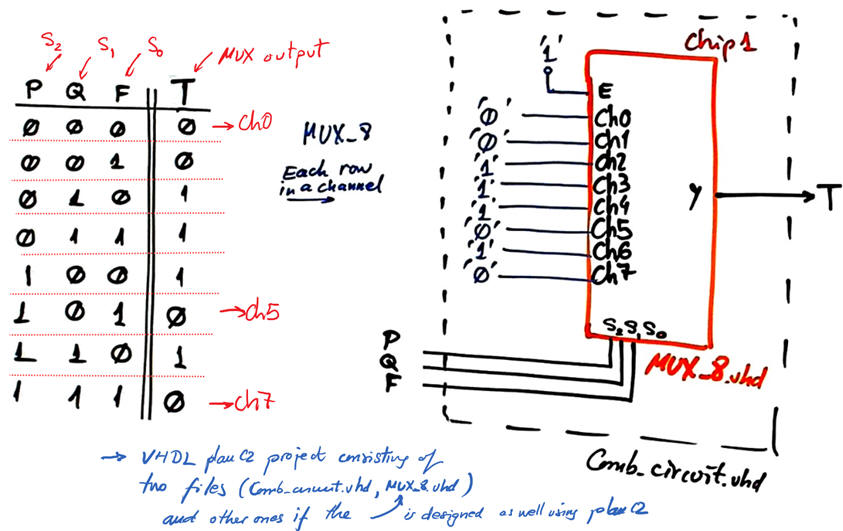Fig. 10. Comb_circuit using MoM and a MUX_8.

Fig 11 shows the solution when designing Comb_circuit using MoM and a MUX_4.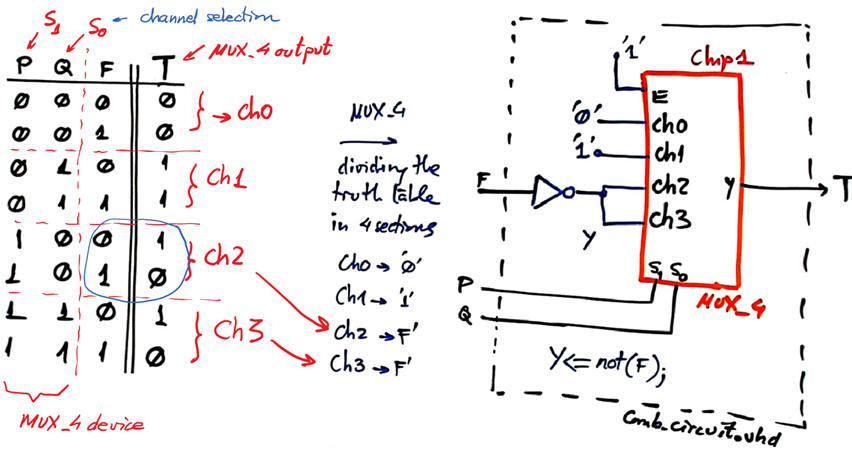Fig. 11. Comb_circuit using MoM and a MUX_4.

Fig 12 shows the solution when designing Comb_circuit using MoM and a MUX_2.Fig. 12. Comb_circuit using MoM and a MUX_2.

A good idea now is to translate to VHDL the schematics in Fig. 10 (Comb_circuit.vhd), Fig. 11  (Comb_circuit.vhd) and Fig 12  (Comb_circuit.vhd), and run synthesis and simulation  (Comb_circuit_tb.vhd) to check whether the circuits generates the truth table in Fig. 9. As usual, place each project in a different folder:

- C:\CSD\P3\Comb_circuit_MoM_MUX_8\(files)

- C:\CSD\P3\Comb_circuit_MoM_MUX_4\(files)

- C:\CSD\P3\Comb_circuit_MoM_MUX_2\(files)

Example design: 3-bit even parity generator using MoM and a MUX_2.Fig. 13. Discussion on how to implement the 3-bit even parity generator using MoM and a MUX_4.

Example design: Circuit_C using MoM and a MUX_4.Fig. 13. Circuit_C symbol and truth table.

A solution using MoM and MUX_4 is represented in Fig. 14.Fig. 14. Proposed solution for Circuit_C

Example design:

- using MoM and MUX_2 (GT), MUX_4 (EQ) and MUX_8 (LT)

Example design:

- 1bit_adder based on the MoM using MUX_2 for So and MUX_4 for Co. .